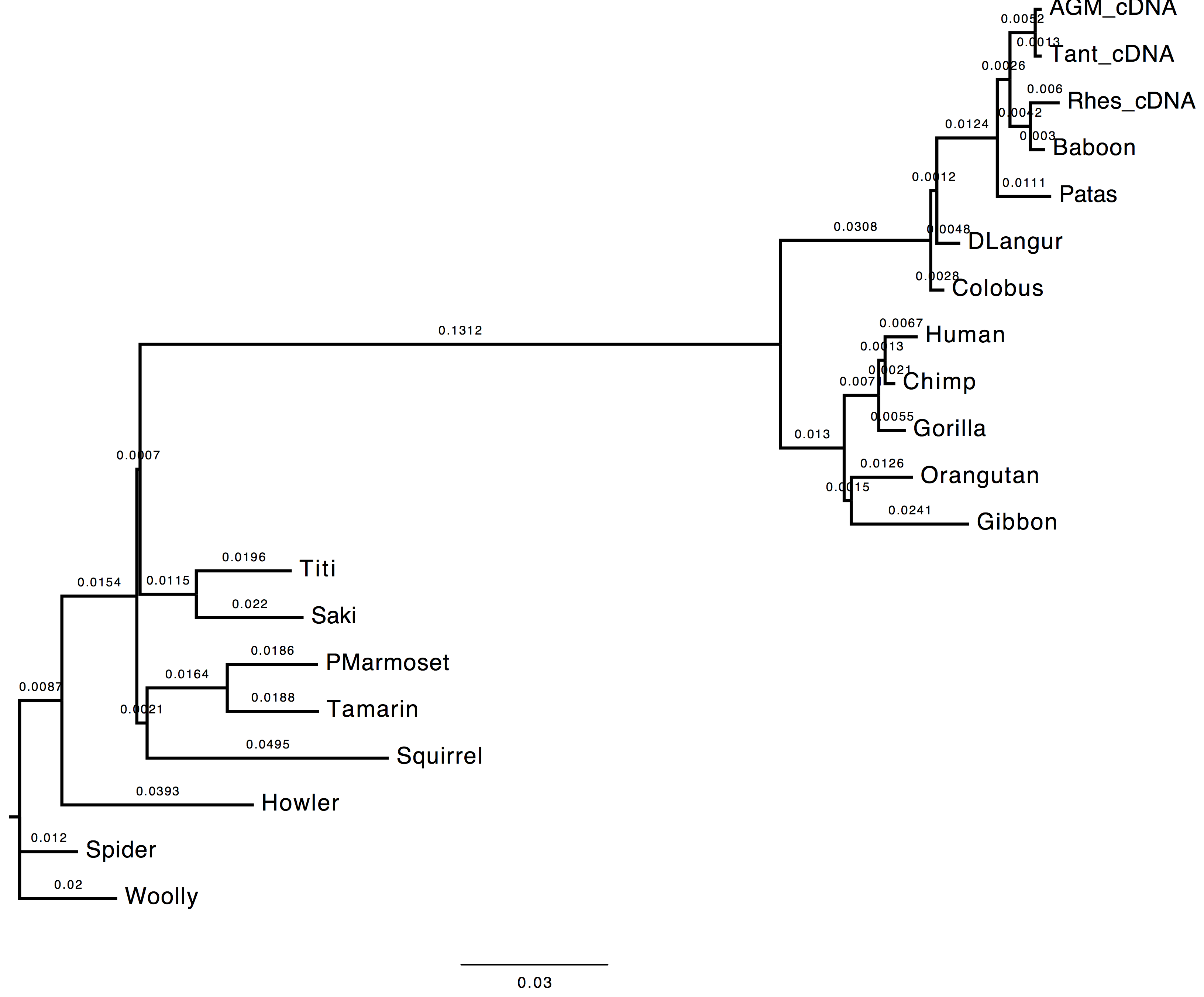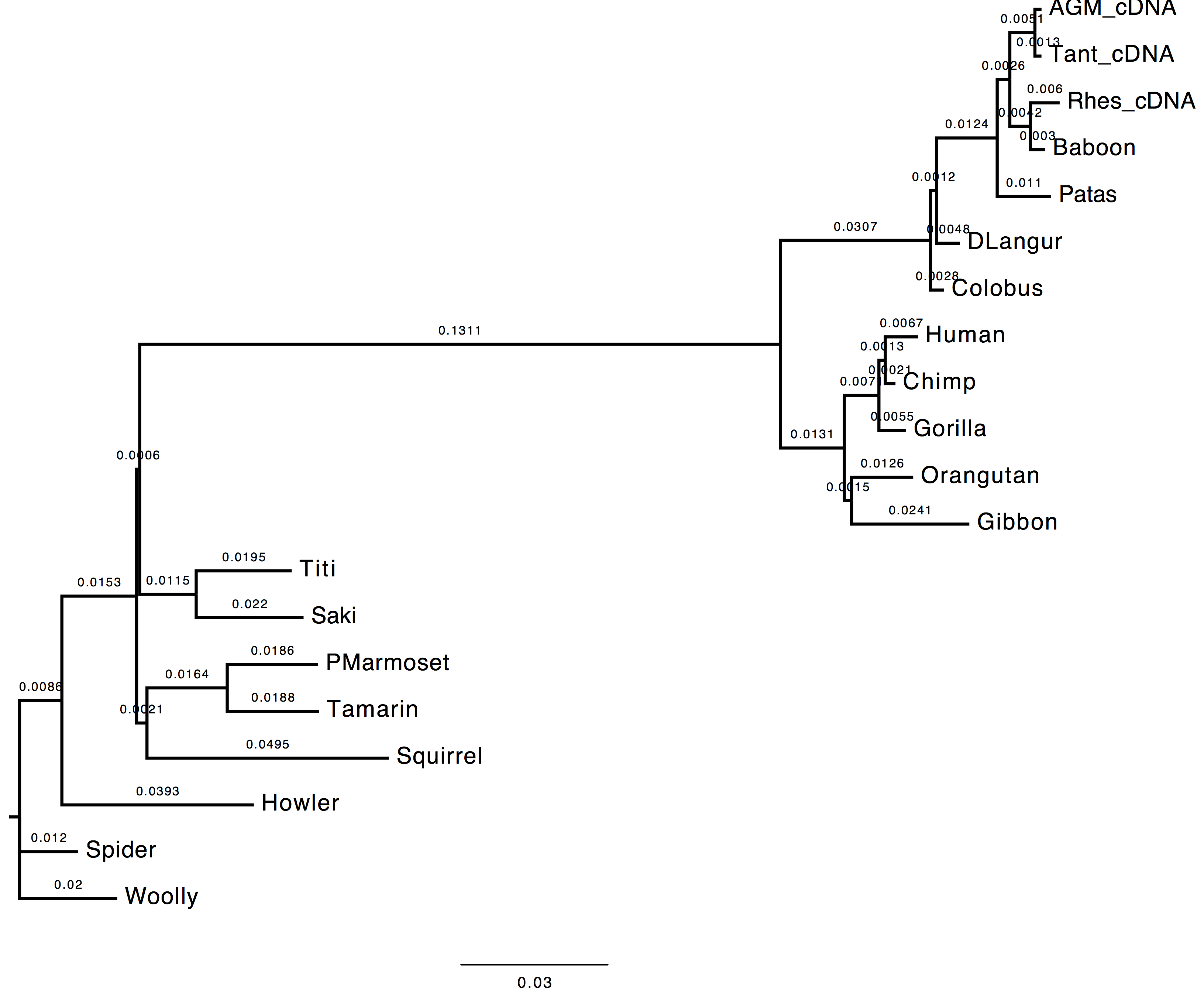# Solution 1 - Parameter estimation

##### Inferring phylogenies using maximum likelihood
###### Observing the effect of parameter estimation from data on the inferred tree topology.

In this exercise you are asked to run PhyML twice in order to compare the effect of estimating nucleotide frequencies from the used dataset vs. optimising them with a maximum likelihood (ML) approach.

Datasets
Dataset file:

##### First Run
• Nucleotide substitution model = HKY85 + Gamma
• Estimating transition/transversion ratio ( $\kappa$ parameter of HKY85 model)
• Estimating alpha parameter (remember $\alpha = \beta$ for gamma distributions used in phylogenetics)
• Estimating nucleotide frequencies with ML

Here is the list of the parameters to change:

[M] ................. Model of nucleotide substitution  HKY85
[F] ................. Optimise equilibrium frequencies  yes
[T] .................... Ts/tv ratio (fixed/estimated)  estimated
[C] ........... Number of substitution rate categories  4
[G] ............. Gamma distributed rates across sites  yes
[A] ... Gamma distribution parameter (fixed/estimated)  estimated


##### Second Run
• Nucleotide substitution model = HKY85 + Gamma
• Estimating transition/transversion ratio ( $\kappa$ parameter of HKY85 model)
• Estimating alpha parameter (remember $\alpha = \beta$ for gamma distributions used in phylogenetics)
• Estimating nucleotide frequencies empirically from the data

Here is the list of the parameters to change:

[M] ................. Model of nucleotide substitution  HKY85
[F] ................. Optimise equilibrium frequencies  no
[T] .................... Ts/tv ratio (fixed/estimated)  estimated
[C] ........... Number of substitution rate categories  4
[G] ............. Gamma distributed rates across sites  yes
[A] ... Gamma distribution parameter (fixed/estimated)  estimated


##### Questions

1. Do you see much difference in the tree?

The topology does not change, however we can observe a change in the computed branch lengths.

Nt-frequencies optimised Nt-frequencies estimated2. In the likelihood value (stat file)?

The likelihood value of the tree inferred using the nucleotide frequencies estimated empirically from the dataset is lower than the likelihood value of the tree inferred using the nucleotide frequencies estimated via ML. This is due to dataset dimensions (the number of the sequences used).

    run 1: -6172.58045
run 2: -6173.49655


3. Which option is best and why do you think so?

The best option in this case is to use the ML approach since the ML optimisation technique provides better estimates for the nucleotide frequencies, which, in turn, affect the likelihood of the inferred tree. This is due to the nucleotide frequencies which are used in the calculation of the likelihood of each site of the alignment ($\pi Q = 0$, where $\pi$ is the vector of the nucleotide frequencies and $Q$ the rate matrix).

phylogenies tree-estimation maximum-likelihood parameter-estimation